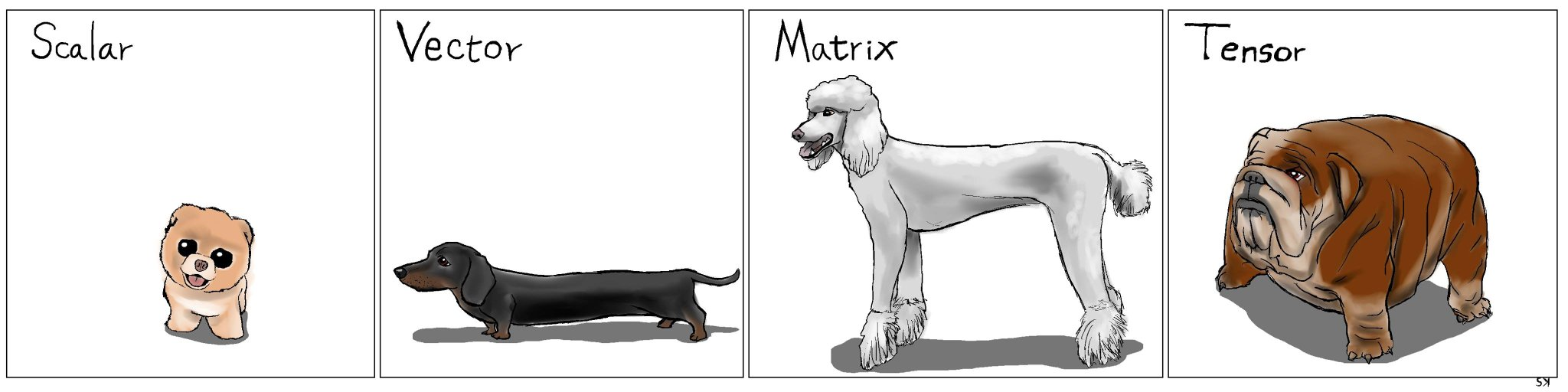# Part 0: Introduction and notation [Draft]

Sait Celebi (celebisait@gmail.com)
Last updated: February 28 2019

"Programming is one of the most difficult branches of applied mathematics; the poorer mathematicians had better remain pure mathematicians." -- Edsger Dijkstra

# Introduction

Welcome to No-magic tutorials!

No-magic tutorials aim to teach Deep Learning fundamentals using first principles with a bottom-up approach.

I call them No-magic tutorials, because I don't like the word "magic". The word "magic" is used arguably commonly in the area of Machine Learning/Deep learning as an escape when failing to understand the actual reasoning behind things. These tutorials attempt to minimize the "magic" portion that prevents the deep understanding of the material. I still constantly go over the tutorials again and think about opportunuties to make it less magic.

The tutorials focus on the underlying math and fundamentals. They generally attempt to derive the necessary ML fundamentals using first principles and apply it with simple Python/Numpy. They, on the other hand, do not try to implement these techniques using highly efficient/parallel data structures. Most of the time, the implementation is close to naive.

# Notation

 $a$ A scalar $\mathbf{a}$ A vector $\mathbf{A}$ A matrix $\mathbf{I}$ An Identity matrix with dimensionality implied by context $\mathbb{A}$ A set $\mathbb{R}$ A set of real numbers $a_i$ Element $i$ of vector $\mathbf{a}$, indexing starting at 1 $A_{i,j}$ Element $(i,j)$ of matrix $\mathbf{A}$ $\mathbf{A_{i,:}}$ Row $i$ of matrix $\mathbf{A}$ $\mathbf{A_{:,i}}$ Column $i$ of matrix $\mathbf{A}$ $\mathbf{A}^T$ Transpose of matrix $\mathbf{A}$ $\mathbf{A} \circ \mathbf{B}$ Element-wise (Hadamard) product of $\mathbf{A}$ and $\mathbf{B}$ $\frac{dy}{dx}$ Derivative of $y$ with respect to $x$ $\frac{\partial y}{\partial x}$ Partial derivative of $y$ with respect to $x$ $\nabla_{\mathbf{x}}y$ Gradient of $y$ with respect to $\mathbf{x}$ $\nabla_{\mathbf{X}}y$ Matrix derivatives of $y$ with respect to $\mathbf{X}$ $\frac{\partial f}{\partial \mathbf{x}}$ Jacobian matrix $\mathbf{J} \in \mathbb{R}^{m \times n}$ of $f: \mathbb{R}^n \rightarrow \mathbb{R}^m$ $P(a)$ A probability distribution over a discrete variable $a$ $p(a)$ A probability distribution over a continuous variable $a$ $f: \mathbb{A} \rightarrow \mathbb{B}$ A function $f$ with domain $\mathbb{A}$ and range $\mathbb{B}$ $f \circ g$ Composition of the functions $f$ and $g$ log $x$ Natural logarithm of $x$ $\mathbf{X}$ An $m \times n$ matrix with input example $\mathbf{x}^{(i)}$ in row $\mathbf{X}_{i,:}$ $\mathbf{x}^{(i)}$ The i-th example (input) from a dataset $\mathbf{y}^{(i)}$ or $y^{(i)}$ The target associated with $\mathbf{x}^{(i)}$ for supervised learning

Or, visually we can summarize our notation as:# Outline of the material

The tutorials are somehow a combination of: (1) Math, (2) Code, (3) Figures and animations. I was using a Jupyter Notebook before, but I wanted more customization over the content and switched to a simple Python script that generates HTML which essentially produces all the tutorials. My HTML generation script, as well as the sources for these tutorials are all in Github, but I do not expect anybody to use those.